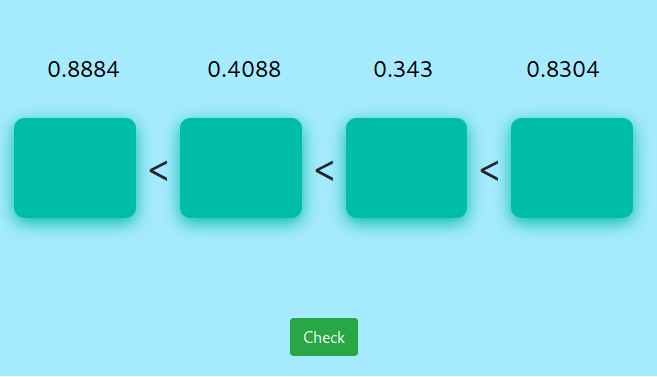MM Practice
×
Multiplication
Division
Place Value
Fractions & Decimals
Measurement
Statistics & Probability
Pre-Algebra
Money
Kindergarten
Geometry

# Order Decimal Numbers — Online Practice Game (grades 4-7)

Practice ordering decimals (from the smallest to the greatest) by dragging them into "boxes" in the correct order. You can choose to order either 3 or 4 decimals.

You can also choose the number of decimal digits for the numbers. For example, students in 4th grade might use decimals with two decimal digits, and students in 5th grade decimals with three decimal digits (places). [Mobile users]: You might have to turn your phone landscape to get the decimals to fit.

Screenshot:Allow my comment to be posted on this site